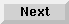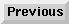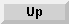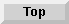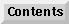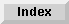2.5 Variable initializations

# 2.5.2 Reference pages

with Variable Initialization Clause Keyword

Syntax:`with` var1 [type-spec] [= expr1] [`and` var2 [type-spec] [= expr2]]*

The`with` construct initializes variables that are local to a loop. The variables are initialized one time only.

If the optional type-spec argument is specified for the variable var, but there is no related expression to be evaluated, var is initialized to an appropriate default value for its data type. For example, for the data types`t`,`number`, and`float`, the default values are`nil`, 0, and 0.0 respectively. It is an error to specify a type-spec argument for var if the related expression returns a value that is not of the specified type.

The optional`and` clause forces parallel rather than sequential initializations.

```;; These bindings occur in sequence.
> (loop with a = 1
with b = (+ a 2)
with c = (+ b 3)
return (list a b c))
(1 3 6)

;; These bindings occur in parallel.
> (setq a 5 b 10)
10

> (loop with a = 1
and b = (+ a 2)
and c = (+ b 3)
return (list a b c))
(1 7 13)

;; This example shows a shorthand way to declare local variables
;; that are of different types.
> (loop with (a b c) (float integer float)
return (format nil "~A ~A ~A" a b c))
"0.0 0 0.0"

;; This example shows a shorthand way to declare local variables
;; that are the same type.
> (loop with (a b c) float
return (format nil "~A ~A ~A" a b c))
"0.0 0.0 0.0"

```

The Loop Facility - 9 SEP 1996Generated with Harlequin WebMaker